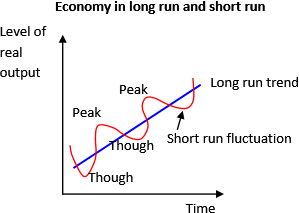## Quiz 6 : An Introduction to MacroeconomicsLooking for Economics Homework Help?# Quiz 6 : An Introduction to Macroeconomics

The average growth rate of Econoland over the 5 years is calculated below:Thus, the average growth rate of Econoland over the five years is 0.6%. In year 3, growth rate is -2% and this situation in the economy is terms as recession. When growth is lower than the average growth rate it is called as recession. If in year 3, growth rate iS2%, then, the average growth rate is calculated below:Thus, the new growth rate iS3 percent.

The graph below shows how economy behaves in long run and short run.In the long run, economy has an uptrend growth. In the short run, there are fluctuations around the long-run trend. When economy fluctuates from peak to trough, it experiences recession; when economy fluctuates from trough to peak, it experiences expansion. Economic fluctuations and economic growth are compatible concepts because economic fluctuations are centered on economic growth. Economic growth is the trend of economic fluctuations.

Change of real output measures the change in output without getting affected by price change. It uses the price of base year to calculate output values.Thus, real output increased by 100% i.e. real output doubled. Real output eliminates the effect of price change, thus, it would still have increasE100% if the price had not changed.